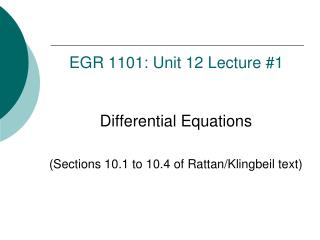DownloadDownload PresentationEGR 1101: Unit 12 Lecture #1

# EGR 1101: Unit 12 Lecture #1

Télécharger la présentation## EGR 1101: Unit 12 Lecture #1

- - - - - - - - - - - - - - - - - - - - - - - - - - - E N D - - - - - - - - - - - - - - - - - - - - - - - - - - -
##### Presentation Transcript

1. EGR 1101: Unit 12 Lecture #1 Differential Equations (Sections 10.1 to 10.4 of Rattan/Klingbeil text)

2. Linear ODE with Constant Coefficients • Given independent variable t and dependent variable y(t), a linear ordinary differential equation with constant coefficients is an equation of the formwhere A0, A1, …, An, are constants.

3. Some Examples • Examples of linear ordinary differential equation with constant coefficients:

4. Forcing Function • In the equation the function f(t) is called the forcing function. • It can be a constant (including 0) or a function of t, but it cannot be a function of y.

5. Solving Linear ODEs with Constant Coefficients • Solving one of these equations means finding a function y(t) that satisfies the equation. • You already know how to solve some of these equations, such as • But many equations are more complicated and cannot be solved just by integrating.

6. A Procedure for Solving Linear ODEs with Constant Coefficients • We’ll use a four-step procedure for solving this type of equation: • Find the transient solution. • Find the steady-state solution. • Find the total solution by adding the results of Steps 1 and 2. • Apply initial conditions (if given) to evaluate unknown constants that arose in the previous steps. • See pages 371-372 in Rattan/Klingbeil textbook.

7. Forcing Function = 0? • If the forcing function (the right-hand side of your differential equation) is equal to 0, then the steady-state solution is also 0. • In such cases, you get to skip straight from Step 1 to Step 3!

8. Some Equations that Our Procedure Can’t Handle • Nonlinear differential equations • Partial differential equations • Diff eqs whose coefficients depend on y or t

9. MATLAB Commands • Without initial conditions: >>dsolve('2*Dy + y = 8') • With initial conditions: >>dsolve('2*Dy + y = 8', 'y(0)=5')

10. MATLAB Commands • Without initial conditions: >>dsolve('D2y+5*Dy+6*y=3*t') • With initial conditions: >>dsolve('D2y+5*Dy+6*y=3*t', 'y(0)=0', 'Dy(0)=0')

11. Today’s Examples • Leaking bucket with constant inflow rate and bucket initially empty • Leaking bucket with zero inflow and bucket initially filled to a given level

12. EGR 1101: Unit 12 Lecture #2 First-Order Differential Equations in Electrical Systems (Section 10.4 of Rattan/Klingbeil text)

13. Review: Procedure • Steps in solving a linear ordinary differential equation with constant coefficients: • Find the transient solution. • Find the steady-state solution. • Find the total solution by adding the results of Steps 1 and 2. • Apply initial conditions (if given) to evaluate unknown constants that arose in the previous steps.

14. Forcing Function = 0? • Recall that if the forcing function (the right-hand side of your differential equation) is equal to 0, then the steady-state solution is also 0. • In such cases, you get to skip straight from Step 1 to Step 3.

15. Today’s Examples • Series RC circuit with constant source voltage • First-order low-pass filter

16. Exponentially Saturating Function • A function of the form where K and  are constants, is called an exponentially saturating function. • At t = 0, f(t) = 0. • As t  , f(t)K.

17. Exponentially Saturating Function: Time Constant • In , the quantity  is called the time constant. • The time constant is a measure of how quickly or slowly the function rises. • The greater  is, the more slowly the function approaches its limiting value K.

18. Time Constant Rules of Thumb • For , • When t = , f(t)  0.632  K. (After one time constant, the function has risen to about 63.2% of its limiting value.) • When t = 5  , f(t)  0.993  K. (After five time constants, the function has risen to about 99.3% of its limiting value.) • See next slide for graph.

19. Exponentially Saturating Function: Graph

20. Exponentially Decaying Function • A function of the form where K and  are constants, is called an exponentially decaying function. • At t = 0, f(t) = K. • As t  , f(t) 0.

21. Exponentially Decaying Function: Time Constant • In , the quantity  is called the time constant. • The time constant is a measure of how quickly or slowly the function falls. • The greater  is, the more slowly the function approaches 0.

22. Time Constant Rules of Thumb • For , • When t = , f(t)  0.368  K. (After one time constant, the function has fallen to about 36.8% of its initial value.) • When t = 5  , f(t)  0.007  K. (After five time constants, the function has fallen toabout 0.7% of its initial value.) • See next slide for graph.

23. Exponentially Decaying Function: Graph

24. Low-Pass and High-Pass Filters • A low-pass filter is a circuit that passes low-frequency signals and blocks high-frequency signals. • A high-pass filter is a circuit that does just the opposite: it blocks low-frequency signals and passes high-frequency signals.